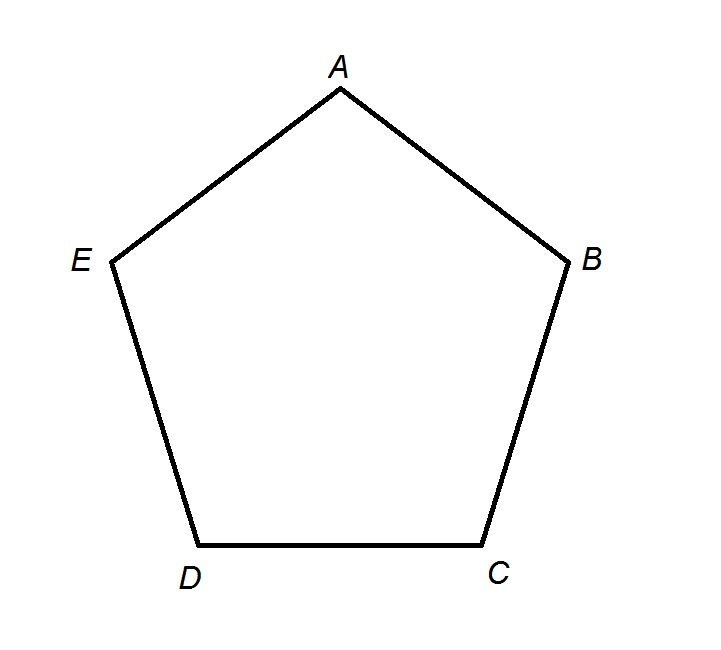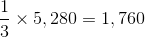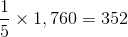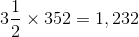## Example Questions

### Example Question #1 : PentagonsThe above diagram shows a pentagonal track with perimeter one third of a mile. Adrianne starts at Point A and runs clockwise until she gets halfway between Points D and E. Which of the following choices is closest to the number of feet she runs?

1,300 feet

1,200 feet

1,100 feet

1,000 feet

1,400 feet

1,200 feet

Explanation:

The perimeter of the pentagonal track is one third of a mile; one mile is equal to 5,280 feet, so the perimeter isfeet.

Each side of the pentagon has length one fifth of its perimeter, orfeet.

Adrianne runs three and one half sides, orfeet.

This makes 1,200 feet the closest, and correct, choice.

### All SAT Math Resources